# Kepler's laws of planetary motion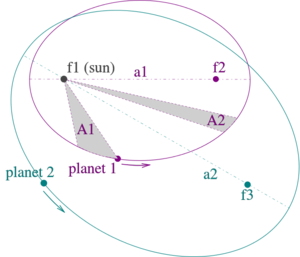Illustration of Kepler's three laws
(1) The orbits are ellipses, with focal points ƒ1 and ƒ2 for the first planet and ƒ1 and ƒ3 for the second planet. The Sun is placed in focal point ƒ1.

(2) The two shaded sectors A1 and A2 have the same area and the time for planet 1 to cover segment A1 is equal to the time to cover segment A2.

(3) The total orbit times for planet 1 and planet 2 have a ratio a13/2 : a23/2.

Kepler's three laws of planetary motion were developed in the early 17th century by Johannes Kepler. Kepler developed the laws after studying the astronomical observations of Tycho Brahe for several years. Kepler realized that the movement of the celestial bodies whose data Brahe had recorded did not fit a circular orbit, as was believed at the time.

Kepler's three laws of planetary motion are:
1. Planets orbit the sun on elliptical orbits with the sun occupying one of the foci of the ellipse.

2. A line connecting the sun to the orbiting body sweeps out an equal area in an equal amount of time regardless of position in the orbit. In other words, the body moves faster when it is closer to the sun and moves slower when it is further away. This law was much later shown to be true because of conservation of angular momentum.

3. The square of the period of the orbit of a body is proportional to the cube of the semi-major axis (the line from one side of the ellipse to the other that passes through both foci) of that orbit.

## Mathematics

### Kepler's Second Law and Conservation of angular momentum

From the diagram, the area swept out, dA, in a very small amount of time dt as the planet moves an angle dθ, can be expressed as: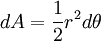Dividing by dt, the rate at which the area is swept out is: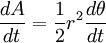Multiplying by the mass of the planet, m, and rearranging: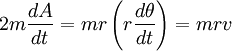where v is the speed of the planet. The right hand side of this equation is the angular momentum of the planet, which must be constant. This means the left hand side must also be constant and so the rate at which area is swept out must bee constant, agreeing with Kepler's second law. From this it can be seen Kepler's second law is due to conservation of angular momentum.

### Kepler's Third Law

For two bodies with masses m1 and m2, Kepler's third law can be expressed as: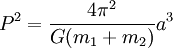Where:

P is the period
G is Newton's gravitational constant
a is the semi-major axis

For bodies in the solar system, if the period is in years and the semi-major axis is in astronomical units, then Kepler's third law may be written more simply as: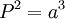as the units incorporate the constant.